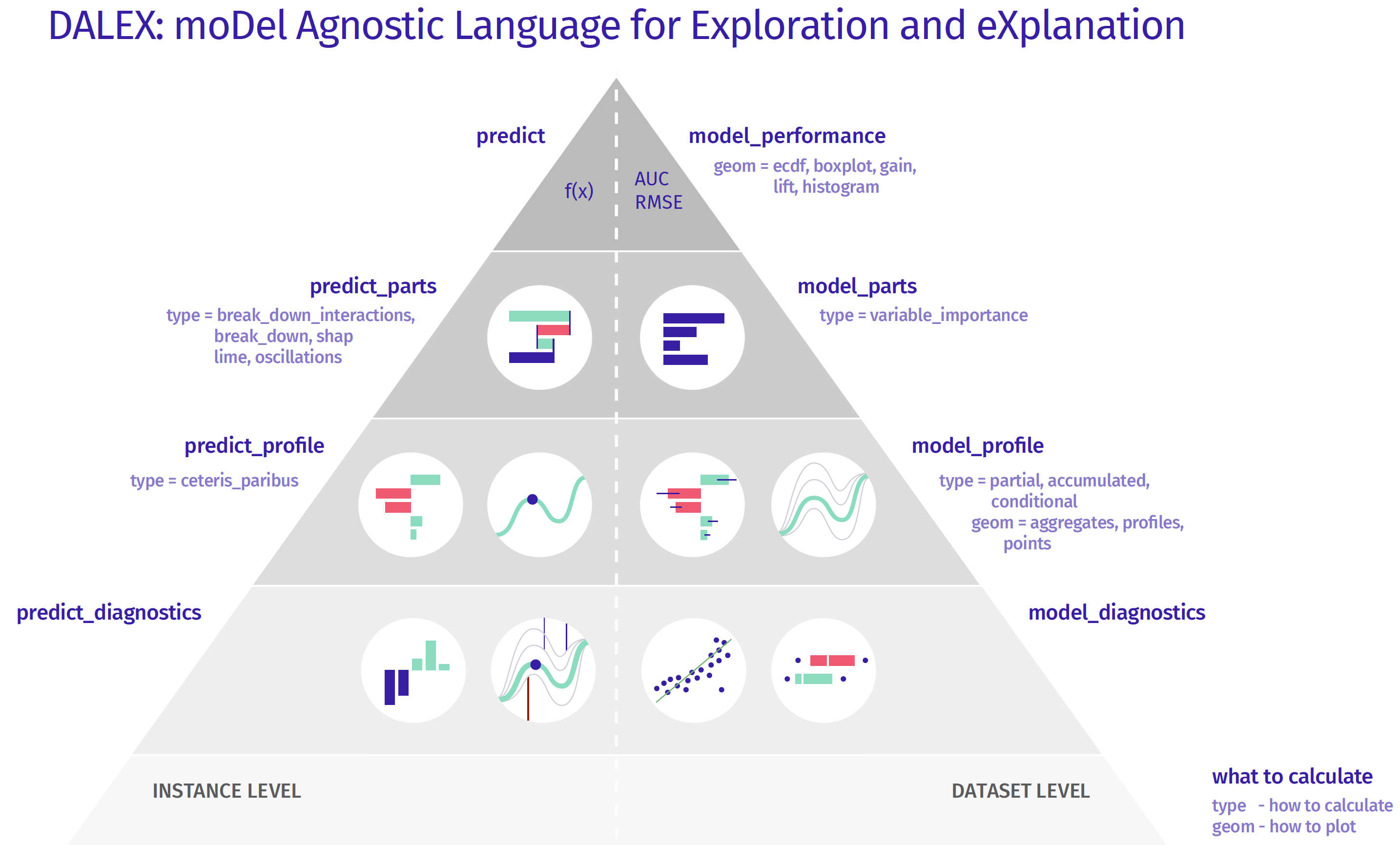# Titanic: tutorial and examples¶

### imports¶

In :
import dalex as dx

import pandas as pd
import numpy as np

from sklearn.neural_network import MLPClassifier
from sklearn.preprocessing import StandardScaler, OneHotEncoder
from sklearn.impute import SimpleImputer
from sklearn.pipeline import Pipeline
from sklearn.compose import ColumnTransformer

import warnings
warnings.filterwarnings('ignore')

In :
dx.__version__

Out:
'0.2.1'

First, divide the data into variables X and a target variable y.

In :
data = dx.datasets.load_titanic()

X = data.drop(columns='survived')
y = data.survived

In :
data.head(10)

Out:
gender age class embarked fare sibsp parch survived
0 male 42.0 3rd Southampton 7.1100 0 0 0
1 male 13.0 3rd Southampton 20.0500 0 2 0
2 male 16.0 3rd Southampton 20.0500 1 1 0
3 female 39.0 3rd Southampton 20.0500 1 1 1
4 female 16.0 3rd Southampton 7.1300 0 0 1
5 male 25.0 3rd Southampton 7.1300 0 0 1
6 male 30.0 2nd Cherbourg 24.0000 1 0 0
7 female 28.0 2nd Cherbourg 24.0000 1 0 1
8 male 27.0 3rd Cherbourg 18.1509 0 0 1
9 male 20.0 3rd Southampton 7.1806 0 0 1

### create a pipeline model¶

• numerical_transformer pipeline:

• numerical_features: choose numerical features to transform
• impute missing data with median strategy
• scale numerical features with standard scaler
• categorical_transformer pipeline:

• categorical_features: choose categorical features to transform
• impute missing data with 'missing' string
• encode categorical features with one-hot
• aggregate those two pipelines into a preprocessor using ColumnTransformer

• make a basic classifier model using MLPClassifier - it has 3 hidden layers with sizes 150, 100, 50 respectively
• construct a clf pipeline model, which combines the preprocessor with the basic classifier model
In :
numerical_features = ['age', 'fare', 'sibsp', 'parch']
numerical_transformer = Pipeline(
steps=[
('imputer', SimpleImputer(strategy='median')),
('scaler', StandardScaler())
]
)

categorical_features = ['gender', 'class', 'embarked']
categorical_transformer = Pipeline(
steps=[
('imputer', SimpleImputer(strategy='constant', fill_value='missing')),
('onehot', OneHotEncoder(handle_unknown='ignore'))
]
)

preprocessor = ColumnTransformer(
transformers=[
('num', numerical_transformer, numerical_features),
('cat', categorical_transformer, categorical_features)
]
)

classifier = MLPClassifier(hidden_layer_sizes=(150,100,50), max_iter=500, random_state=0)

clf = Pipeline(steps=[('preprocessor', preprocessor),
('classifier', classifier)])


### fit the model¶

In :
clf.fit(X, y)

Out:
Pipeline(steps=[('preprocessor',
ColumnTransformer(transformers=[('num',
Pipeline(steps=[('imputer',
SimpleImputer(strategy='median')),
('scaler',
StandardScaler())]),
['age', 'fare', 'sibsp',
'parch']),
('cat',
Pipeline(steps=[('imputer',
SimpleImputer(fill_value='missing',
strategy='constant')),
('onehot',
OneHotEncoder(handle_unknown='ignore'))]),
['gender', 'class',
'embarked'])])),
('classifier',
MLPClassifier(hidden_layer_sizes=(150, 100, 50), max_iter=500,
random_state=0))])

### create an explainer for the model¶

In :
exp = dx.Explainer(clf, X, y)

Preparation of a new explainer is initiated

-> data              : 2207 rows 7 cols
-> target variable   : Argument 'y' was a pandas.Series. Converted to a numpy.ndarray.
-> target variable   : 2207 values
-> model_class       : sklearn.neural_network._multilayer_perceptron.MLPClassifier (default)
-> label             : not specified, model's class short name is taken instead (default)
-> predict function  : <function yhat_proba_default at 0x000002221E2B09D0> will be used (default)
-> model type        : classification will be used (default)
-> predicted values  : min = 2.72e-06, mean = 0.337, max = 1.0
-> predict function  : accepts only pandas.DataFrame, numpy.ndarray causes problems
-> residual function : difference between y and yhat (default)
-> residuals         : min = -0.921, mean = -0.0146, max = 0.975
-> model_info        : package sklearn

A new explainer has been created!


# dalex functions¶Above functions are accessible from the Explainer object through its methods.

Each of them returns a new unique object that contains a result field in the form of a pandas.DataFrame and a plot method.

## INSTANCE LEVEL¶

### predict¶

This function is nothing but normal model prediction, however it uses Explainer interface.

Let's create two example persons for this tutorial.

In :
john = pd.DataFrame({'gender': ['male'],
'age': ,
'class': ['1st'],
'embarked': ['Southampton'],
'fare': ,
'sibsp': ,
'parch': 0},
index = ['John'])

In :
mary = pd.DataFrame({'gender': ['female'],
'age': ,
'class': ['3rd'],
'embarked': ['Cherbourg'],
'fare': ,
'sibsp': ,
'parch': },
index = ['Mary'])


You can make a prediction on many samples at the same time

In :
exp.predict(X)[0:10]

Out:
array([0.07907226, 0.20628711, 0.13463174, 0.60372994, 0.76485216,
0.16150944, 0.03705073, 0.99324938, 0.19563509, 0.12184964])

As well as on only one instance. However, the only accepted format is pandas.DataFrame.

Prediction of survival for John.

In :
exp.predict(john)

Out:
array([0.08127727])

Prediction of survival for Mary.

In :
exp.predict(mary)

Out:
array([0.8929144])

### predict_parts¶

• 'break_down'

• 'break_down_interactions'

• 'shap'

This function calculates Variable Attributions as Break Down, iBreakDown or Shapley Values explanations.

Model prediction is decomposed into parts that are attributed for particular variables.

In :
bd_john = exp.predict_parts(john, type='break_down')
bd_interactions_john = exp.predict_parts(john, type='break_down_interactions')

sh_mary = exp.predict_parts(mary, type='shap', B = 10)

In :
bd_john.result.label = "John"
bd_interactions_john.result.label = "John+"

bd_john.result

Out:
variable_name variable_value variable cumulative contribution sign position label
0 intercept 1 intercept 0.336735 0.336735 1.0 8 John
1 class 1st class = 1st 0.583093 0.246358 1.0 7 John
2 age 25.0 age = 25.0 0.595401 0.012308 1.0 6 John
3 sibsp 0.0 sibsp = 0.0 0.585751 -0.009650 -1.0 5 John
4 fare 72.0 fare = 72.0 0.319029 -0.266722 -1.0 4 John
5 parch 0.0 parch = 0.0 0.300772 -0.018257 -1.0 3 John
6 embarked Southampton embarked = Southampton 0.284191 -0.016580 -1.0 2 John
7 gender male gender = male 0.081277 -0.202914 -1.0 1 John
8 prediction 0.081277 0.081277 1.0 0 John
In :
bd_john.plot(bd_interactions_john)